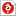검색어 입력폼

# Data Structures HW2

저작시기 2011.04 |등록일 2012.12.01한컴오피스 (hwp) | 2페이지 | 가격 2,000원

## 소개글

연세대학교 데이타구조 숙제입니다. 만점받았습니다

## 목차

(1) Brief explanation of the problem
(2) Your view as to how it ties in with what we covered in class
(4) Running time analysis and discussion
(5) Merits and drawbacks of recursive functions

## 본문내용

(1) Brief explanation of the problem
- There are many kinds of algorithm analysis. Especially, Space complexity and Time complexity is classic. By the way, time complexity is more important than space, because space available tends to be larger and larger but time don`t. So ,in this present homework, we are only interested in running time. The running time is different according to a code. Therefore it is a goal to find out my running time and analysis my code and finally think about my merits and drawbacks of my code.
(2) Your view as to how it ties in with what we covered in class
- We learned about algorithm analysis. The running time means if the algorithm is fast or not. Most of all the Asymptotic Notation which is to simplify analysis by getting rid of unneeded information and Big-Oh Notation is related with this homework.

<중 략>

(4) Running time analysis and discussion
- In solving the running time, algorithms are measured by their worst case typically. And the function call must be analyzed first.
So ,in my code, I consider the function call(line7~10) first.
when n=0 , line 8 is true so the running time is k(arbitrary constant).
when n>0, line 9 and 10 is worked. So if we assume T(n) is the running time fibo(n) then we can find the running time is T(n)=T(n-1)+k` where k` include line 8 , % and +(or/). By an arithmetical progression, T(n)=3n+k``(k`` is arbitrary constant).
Lastly, we should consider for function(line4). In line 4 the running time is 2m+4 because of 1(i=0)+(m+2(i?n))+(m+1(i++)).

## 참고 자료

Data Structures and Algorithm Analysis In C (Mark Allen Weiss)